# JP4552635B2 - Multi-carrier transmission apparatus and multi-carrier transmission method - Google Patents

## Info

Publication number
JP4552635B2
JP4552635B2 JP2004354004A JP2004354004A JP4552635B2 JP 4552635 B2 JP4552635 B2 JP 4552635B2 JP 2004354004 A JP2004354004 A JP 2004354004A JP 2004354004 A JP2004354004 A JP 2004354004A JP 4552635 B2 JP4552635 B2 JP 4552635B2
Authority
JP
Japan
Prior art keywords
snr
value
minimum
bit allocation
transmission
Prior art date
Legal status (The legal status is an assumption and is not a legal conclusion. Google has not performed a legal analysis and makes no representation as to the accuracy of the status listed.)
Expired - Fee Related
Application number
JP2004354004A
Other languages
Japanese (ja)
Other versions
JP2006165978A (en
Inventor

Original Assignee

Priority date (The priority date is an assumption and is not a legal conclusion. Google has not performed a legal analysis and makes no representation as to the accuracy of the date listed.)
Filing date
Publication date
Application filed by 日本電気株式会社 filed Critical 日本電気株式会社
Priority to JP2004354004A priority Critical patent/JP4552635B2/en
Publication of JP2006165978A publication Critical patent/JP2006165978A/en
Application granted granted Critical
Publication of JP4552635B2 publication Critical patent/JP4552635B2/en
Application status is Expired - Fee Related legal-status Critical
Anticipated expiration legal-status Critical

## Images

•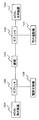•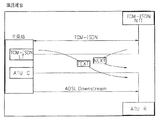••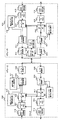•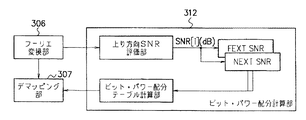•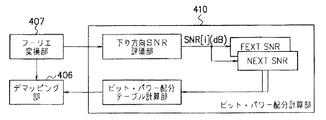•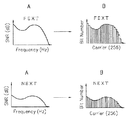•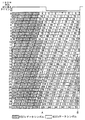•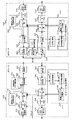••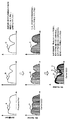•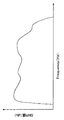•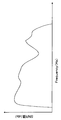•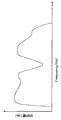•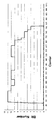••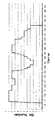•••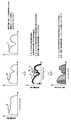•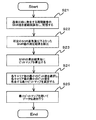•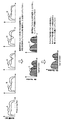••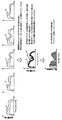•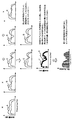•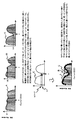••## Classifications

• HELECTRICITY
• H04ELECTRIC COMMUNICATION TECHNIQUE
• H04LTRANSMISSION OF DIGITAL INFORMATION, e.g. TELEGRAPHIC COMMUNICATION
• H04L5/00Arrangements affording multiple use of the transmission path
• H04L5/003Arrangements for allocating sub-channels of the transmission path
• H04L5/0058Allocation criteria
• H04L5/006Quality of the received signal, e.g. BER, SNR, water filling
• HELECTRICITY
• H04ELECTRIC COMMUNICATION TECHNIQUE
• H04LTRANSMISSION OF DIGITAL INFORMATION, e.g. TELEGRAPHIC COMMUNICATION
• H04L5/00Arrangements affording multiple use of the transmission path
• H04L5/003Arrangements for allocating sub-channels of the transmission path
• H04L5/0044Arrangements for allocating sub-channels of the transmission path allocation of payload
• H04L5/0046Determination of how many bits are transmitted on different sub-channels
• HELECTRICITY
• H04ELECTRIC COMMUNICATION TECHNIQUE
• H04LTRANSMISSION OF DIGITAL INFORMATION, e.g. TELEGRAPHIC COMMUNICATION
• H04L5/00Arrangements affording multiple use of the transmission path
• H04L5/0001Arrangements for dividing the transmission path
• H04L5/0003Two-dimensional division
• H04L5/0005Time-frequency
• H04L5/0007Time-frequency the frequencies being orthogonal, e.g. OFDM(A), DMT

## Description

The present invention is a multi-carrier applied to xDSL (x Digital Subscriber Line) (x is a generic name of A, S, V, etc.) that enables high-speed data transmission of several Mbit / s over a metallic cable such as a telephone line. More particularly, the present invention relates to a multicarrier transmission apparatus and a multicarrier transmission method that perform data transmission in a noise environment in which sudden noise occurs.

In recent years, attention has been focused on xDSL technology that enables high-speed data transmission of several megabits / second using a metallic cable such as a telephone line. Among them, ADSL (Asymmetric Digital Subscriber Line) is attracting attention. This ADSL has different transmission speeds between upstream and downstream, and this asymmetry is suitable for Internet access.

First, a system configuration of a general ADSL transmission system will be described with reference to FIG.

As shown in FIG. 1, the ADSL transmission system includes an ADSL in-home device (100), a home phone (101), a splitter (102) inside the home, an ADSL in-house device (104), and a telephone switch (105). , And a splitter (106) inside the station.

The ADSL in-home device (100) is connected to the line (103) via a splitter (102) inside the home. The home phone (101) is connected to the line (103) via the splitter (102) on the inside of the home.

The ADSL intra-station device (104) is connected to the line (103) via the splitter (106) inside the station. The telephone exchange (105) is connected to the line (103) via the splitter (106) inside the office.

The splitter (102, 106) is a device for separating a signal in the line (103) into a speech signal and a data signal by ADSL, and the splitter (102) inside the house is in the line (103). Is connected to the home phone (101) side when the signal is a call signal, and is connected to the ADSL home device (100) side when the signal in the line (102) is a data signal by ADSL. It will be. Further, the splitter (106) inside the station is connected to the telephone exchange (105) when the signal in the line (103) is a call signal, and the signal in the line (103) is an ADSL signal. Is connected to the ADSL in-station device (104) side. The ADSL intra-station apparatus (104) has a DSLAM (Digital Subscriber Line Multiplexer), and is connected to the Internet from the provider via the DSLAM. Note that the DSLAM converts data transmitted as an analog signal into a digital signal and transmits it to a provider.

The ADSL transmission system converts a digital signal into an analog signal using a modulation / demodulation method called DMT (Discrete Multi-Tone), and transmits the analog signal.

In the DMT scheme, modulation processing by QAM (Quadrature Amplitude / Phase Modulation) is performed on 256 carriers, and the modulated carriers are multiplexed and transmitted using inverse Fourier transform. The receiving side extracts each carrier using Fourier transform based on the multiplexed signal, performs demodulation processing on the QAM-modulated signal, and performs high-speed data transmission.

However, in this DMT ADSL transmission system, if the ADSL cable is included in the same cable bundle as the ISDN cable, noise that reduces the communication speed of the ADSL line due to the influence of the ISDN cable. There will be a factor that generates. Among them, the crosstalk noise from the ISDN line has a great influence on the ADSL line. Therefore, it is possible to avoid the generation of noise by configuring the ISDN line and the ADSL line so that they are not included in the same cable bundle. However, in this case, the burden on the operator is too great. Therefore, a transmission method capable of avoiding a decrease in communication speed due to crosstalk noise generated when an ISDN line and an ADSL line are included in a bundle in the same cable, and ensuring a transmission amount. Is desired.

First, crosstalk noise generated in an ADSL apparatus when a TCM ISDN line is used will be described with reference to FIG. In FIG. 2, when data is transmitted in the downlink direction, it is generated in an ATU-R (ADSL Transceiver Unit-Remote side), which is a device on the terminal side of the ADSL device, by data transmission through the TCM-ISDN line. Crosstalk noise is shown.

In the TCM ISDN line, data transmission in the upstream direction and the downstream direction is alternately performed every 1.25 msec. When the TCM-ISDN line performs uplink data transmission while performing downlink data transmission on the ADSL line, the high-power signal before attenuation from the TCM-ISDN line is attenuated on the ADSL line. This affects the signal and causes “NEXT” (Near End Cross Talk) and “Near End Crosstalk” to occur on the terminal ATU-R side.

In addition, when the TCM-ISDN line performs downlink data transmission while performing downlink data transmission on the ADSL line, the TCM-ISDN line signal affects the attenuated ADSL line signal, "FEXT" (Far End Cross Talk) and "far end crosstalk" will occur on the terminal side ATU-R side. The same phenomenon also occurs in an ATU-C (ADSL Transceiver Unit-Center Side) which is a device on the central office side.

Next, the amount of crosstalk noise will be described with reference to FIG. FIG. 3 shows the amount of crosstalk noise. As shown in FIG. 3, the noise amount of “NEXT” when near-end crosstalk occurs is larger than the noise amount of “FEXT” when far-end crosstalk occurs. This is because the high-power signal before attenuation from the TCM-ISDN line affects the attenuated signal on the ADSL line. Paying attention to the difference in the amount of noise, a method has been proposed in which the transmission amount of data is switched between “NEXT” when near-end crosstalk occurs and “FEXT” when far-end crosstalk occurs. This method is called a dual bitmap method. As shown in FIG. 3, when a far-end crosstalk “FEXT” having a noise amount smaller than a predetermined threshold occurs, a large amount of data is transmitted, and the noise amount is a predetermined threshold value. When more near-end crosstalk “NEXT” occurs, a smaller amount of data is transmitted.

As described above, in the ADSL transmission system in which the TCM ISDN line and the ADSL line are adjacent to each other, the amount of noise periodically changes. Therefore, the SNR (Signal to Noise Ratio) of each carrier is increased in the uplink direction and the downlink direction. The bit allocation is determined according to the measured SNR.

Next, a conventional ADSL transmission system will be described with reference to FIG.

<ATU-C300 side configuration>
First, the configuration on the ATU-C (300) side will be described.

The transmission unit of the ATU-C (300) includes a CRC error processing unit (315) that adds a CRC (Cyclic Reliability Check) code to data sent from the host device, and a data that has the CRC code added thereto. The scramble process and error correction unit (scram & FEC (Forward Error Correction)) (301) for applying a scramble process and adding a Reed-Solomon error correction code, and the transmission power of each carrier according to the timing at which the noise level changes A mapping unit (302) that switches between allocation and bit allocation and performs bit allocation and transmission power allocation to a carrier, and a multilevel QAM (Quadrature Amplitude Modulation) signal that is the mapping output Inverse Fourier transform unit for modulating multiplexed with a carrier and (303), and the multiplexed output has a digital / analog converter for transmitting the analog data as a downlink analog signal (304), the.

The ATU-C (300) receiving unit includes an analog / digital conversion unit (305) that converts an analog signal transmitted from the ATU-R (400) into a digital signal, and performs a Fourier transform on the digital signal. A Fourier transform unit (306), a demapping unit (307) that demodulates the transmitted signal by switching between bit distribution and transmission power distribution according to the timing of changing the noise level, and scrambles and performs error correction Using the scramble process and error correction unit (scram & FEC (Forward Error Correction)) (308) that performs processing to return to the correct data by the above and a predetermined formula, the CRC code added to the data is checked, CRC error detection unit (314) that performs error detection is doing.

The ATU-C (300) is provided with a pseudo-random signal generator (310), a noise tone generator (311), and a bit power distribution calculator (312). FIG. 5 shows a detailed configuration of the bit power distribution calculation unit (312).

<ATU-R400 side configuration>
Next, the configuration on the ATU-R (400) side will be described.

The transmission unit of the ATU-R (400) includes a CRC error processing unit (415) for adding a CRC (Cyclic Reliability Check) code to data sent from the host device, and a data for which the CRC code is added. Scramble processing and error correction unit (scram & FEC (Forward Error Correction)) (401) that performs scramble processing and adds a Reed-Solomon error correction code, and the transmission power of each carrier according to the timing at which the noise level changes A mapping unit (402) that performs bit allocation and transmission power allocation to the carrier by switching between allocation and bit allocation, and a Fourier inverse transform unit (403) that modulates and multiplexes the multilevel QAM signal that is the mapping output on each carrier; , This multiplexed output Analog of digital / analog conversion unit to be transmitted as an uplink signal and (404), a has.

The ATU-R (400) receiving unit has an analog / digital conversion unit (408) that converts an analog signal transmitted from the ATU-C (300) into a digital signal, and performs a Fourier transform on the digital signal. A Fourier transform unit (407), a demapping unit (406) that demodulates the transmitted signal by switching between bit distribution and transmission power distribution according to the timing of changing the noise level, and scrambles and performs error correction Using the scramble process and error correction unit (scram & FEC (Forward Error Correction)) (405) that performs processing for returning to the correct data by the above and a predetermined formula, the CRC code added to the data is checked, CRC error detection unit (414) for error detection is doing.

The ATU-R (400) is provided with a pseudo random signal generation unit (409) and a bit power distribution calculation unit (410). FIG. 6 shows a detailed configuration of the bit power distribution calculation unit (410).

In the ADSL transmission system shown in FIG. 4, near-end crosstalk “NEXT” occurs in ATU-C (300) and far-end crosstalk “FEXT” occurs in ATU-R (400) during downstream transmission of ISDN. become. Further, when ISDN is transmitted in the upstream direction, far end crosstalk “FEXT” occurs in ATU-C (300), and near end crosstalk “NEXT” occurs in ATU-R (400).

The pseudo-random signal generator (310, 409) sequentially assigns data forming a predetermined pseudo-random sequence to each carrier used for data transmission in order to secure the data transmission capacity in the above-described noise environment. Pseudo-random signals are generated and output to the inverse Fourier transform units (303, 403), respectively, and output to the opposite station side via the digital / analog conversion units (304, 404).

The bit power allocation calculation unit (312, 410) is configured to allocate a bit allocation to each carrier used for data transmission using the pseudo random signal generated by the pseudo random signal generation unit (409, 310) on the opposite station side, and The transmission power distribution to be used for the carrier is determined when near-end crosstalk occurs and when far-end crosstalk occurs. Then, the bit power allocation calculation unit (312 and 410) calculates the bit allocation and the transmission power allocation obtained when the near end crosstalk occurs and when the far end crosstalk occurs, respectively, and the demapping unit (307) on the local station side. , 406) and the opposite station side mapping section (302, 402).

Next, processing operations when the bit power distribution calculation unit (312, 410) obtains bit distribution and transmission power distribution will be described. Since the ATU-C (300) and the ATU-R (400) perform the same process, only the process for obtaining the bit distribution in the downlink direction and the transmission power distribution will be described below.

First, the pseudo random signal generation unit (310) predetermines the amplitude of each carrier used for data transmission in the training period for calculating the bit allocation to be assigned to the carrier and the transmission power allocation to be used for each carrier. Modulation is performed according to the sequence of bits of predetermined data assigned according to the pseudo-random sequence, and the modulated amplitude of each carrier is output to the inverse Fourier transform unit (303).

The Fourier inverse transform unit (303) performs an inverse Fourier transform on each carrier whose amplitude is modulated, and outputs a voltage value expressed in a digital format obtained by adding the carriers. The digital / analog converter (304) converts the digital voltage value into an analog signal that is an actual voltage value and outputs the analog signal to the line.

The ATU-R (400) converts the analog signal sent from the ATU-C (300) into a voltage value represented in a digital format by the analog / digital conversion unit (408). The Fourier transform unit (407) performs Fourier transform on the digital voltage value to extract each carrier whose amplitude is modulated.

Each carrier extracted by the Fourier transform unit (407) is output to the bit power distribution calculation unit (410).

The bit power allocation calculation unit (410) calculates a plurality of SNR values for each carrier at the time of NEXT generation and at the time of FEXT generation by the downlink SNR evaluation unit, and calculates an average value of SNR of each carrier. .

Note that A shown in FIG. 7 indicates the average value of the SNR when the FEXT occurs and the average value of the SNR when the NEXT occurs, which are evaluated by the downlink SNR evaluation unit.

The downlink SNR evaluation unit shown in FIG. 6 holds the calculated SNR average value when NEXT occurs in NEXT SNR and the SNR average value when FEXT occurs in FEXT SNR, respectively.

Further, the bit power distribution calculation unit calculates the bit distribution and transmission power distribution of each carrier for each noise level based on the measured SNR average value of each carrier, and calculates the calculated bit distribution and transmission power distribution. The data is output to the demapping unit (406) and stored, and also output to the mapping unit (402). Note that B shown in FIG. 7 conceptually shows a state in which the bit allocation of each carrier is determined according to the average value of the SNR evaluated by the downlink SNR evaluation unit.

The mapping unit (402) uses the bit allocation calculated by the bit power allocation calculation unit (410) during the training period for calculating the bit allocation allocated to the carrier used for data transmission and the transmission power allocation used for the carrier, and The transmission power distribution information is allocated to a predetermined carrier by a predetermined number of bits, and the allocated bit distribution and transmission power distribution information are output to the inverse Fourier transform unit (403).

The inverse Fourier transform unit (403) performs inverse Fourier transform on the predetermined carrier sent from the mapping unit (402), and outputs a voltage value expressed in digital format. The digital / analog conversion unit (404) generates an analog signal that is an actual voltage value based on the voltage value expressed in a digital format, and outputs the analog signal to the line.

The ATU-C (300) converts the analog signal sent from the ATU-R (400) into a voltage value represented in a digital format by the analog / digital conversion unit (305). Then, the Fourier transform unit (306) performs Fourier transform on the digital voltage value, and takes out each carrier whose amplitude is modulated.

The demapping unit (307) extracts information on bit distribution and transmission power distribution from a predetermined carrier assigned by a predetermined number of bits, and maps the extracted bit distribution and transmission power distribution information on the mapping unit ( 302) and stored.

The mapping unit (302, 402) uses the two types of bit allocation and transmission power allocation calculated by the above processing to perform bit allocation and transmission power allocation according to the noise level generated during data transmission. Select and perform bit allocation and transmission power allocation to each carrier. Further, the demapping units (307, 406) are allocated to the carrier by using the same bit allocation and transmission power allocation as the bit allocation and transmission power allocation made according to the noise level in the opposite station. Data will be retrieved.

The ADSL transmission system shown in FIG. 4 has a noise synchronization tone generator (311) on the ATU-C (300) side, and a clock detector (411) on the ATU-R (400) side. ) And a bit power distribution selection unit (412).

The clock on the ATU-C (300) side is a clock synchronized with the timing at which the noise level changes, and in this case, the timing at which the noise level changes is known. For example, when the noise is crosstalk from a TCM ISDN line, near-end crosstalk and far-end crosstalk occur every 1.25 msec, so that the SNR of each carrier also changes every 1.25 msec. Therefore, the transmission unit of the ATU-C (300) receives a clock that changes in amplitude at a period of 1.25 msec synchronized with the timing at which the noise level changes, and transmits the clock to the reception unit of the ATU-R (400). It will be necessary. Therefore, a noise synchronization tone generator (311) generates a noise synchronization tone signal whose signal level is changed in synchronization with the clock and transmits it to the ATU-R (400). More specifically, the noise synchronization tone generation unit (311) changes the amplitude of a predetermined carrier in synchronization with the timing at which the noise level changes by a clock synchronized with the timing at which the noise level changes, and performs inverse Fourier transform. Part (303).

The clock detection unit (411) detects the timing at which the noise level changes due to the change in the amplitude of the predetermined carrier extracted by the Fourier transform unit (407), and distributes the detected timing at which the noise level changes to the bit power distribution. It transmits to a selection part (412).

The bit power allocation selection unit (412) recognizes the timing at which the noise level changes in response to the notification from the clock detection unit (411), and stores the two types of bit allocations stored in the mapping unit (402) and the transmission power. Among the distributions, the bit distribution used to perform data transmission according to the noise level and the transmission power distribution are specified. The bit power distribution selection unit (412) uses the ATU-C (300) for noise demodulation among the two types of bit distributions and transmission power distributions stored in the demapping unit (406). The bit allocation used in accordance with the level and the same bit allocation and transmission power allocation as the transmission power allocation are designated.

FIG. 8 shows the configuration of a hyperframe composed of 345 symbols. The symbol on the left side of the dotted line A shown in FIG. 8 is a symbol that has low crosstalk noise from the ISDN line (far-end crosstalk occurs) and can allocate many bits to the carrier. In addition, the symbol between the dotted lines A and B shown in FIG. 8 is a symbol that has a large amount of crosstalk noise from the ISDN line (far-end crosstalk occurs) and can allocate only a few bits to the carrier.

When transmission is started from the 0th symbol in synchronization with the far-end crosstalk generation timing from the ISDN, the reception timing of the 345th symbol and the timing of switching of the crosstalk noise from the ISDN are synchronized as shown in FIG. . Therefore, it is possible to transmit symbols in synchronization with the far-end crosstalk occurrence timing from ISDN from the next 346th symbol. The bit power distribution selection unit (412) stores which bit distribution and transmission power distribution to use among the two types of bit distribution and transmission power distribution for each symbol transmission order. Has been.

The inverse Fourier transform unit (303) outputs signals from the pseudo random signal generation unit (310), the noise synchronization tone generation unit (311), and the mapping unit (302). Signals output from the apparatus are not simultaneously input to the inverse Fourier transform unit (303). That is, the inverse Fourier transform unit (303) performs inverse Fourier transform on signals input at different times and outputs the result to the digital / analog conversion unit (304). Each device described above is controlled by a sequencer (not shown). Under the control of the sequencer, the pseudo random signal generator (310) and the noise synchronization tone generator (311) output signals to the inverse Fourier transform unit (303) when the predetermined signal output timing is reached. The Fourier inverse transform unit (303) recognizes from which device the signal is input next by the sequencer.

In addition, the crosstalk noise from the TCM-ISDN existing in the adjacent line is generated as shown in FIG. 3 with the far end crosstalk “FEXT” and the near end crosstalk “NEXT” every 400 Hz, and the noise periodicity is 400 Hz. Therefore, in the conventional ADSL transmission system, it is possible to predict the period of crosstalk noise from the TCM-ISDN using a 400 Hz clock and avoid errors due to periodically generated noise. Met.

However, there is a problem that burst noise occurs during communication for a short time, and the line connection is disconnected. In a conventional ADSL transmission system, when a short burst noise occurs during communication due to various external factors, a PSD (Power Spectrum Density) or period of the short burst noise is predicted. In the normal initialization / training time, the PSD cannot be measured and the bit allocation used for data transmission becomes inaccurate. For this reason, when irregular periodic noise occurs, it is difficult to perform multicarrier transmission efficiently.

In addition, as a technical document filed prior to the present invention, the transmission power distribution of each carrier of the multicarrier is calculated according to the period of periodically changing noise, and data transmission is performed based on the calculated transmission power distribution. In some cases, multi-carrier transmission is efficiently performed in a state where periodically changing noise is generated (see, for example, Patent Document 1).

In addition, there is one that performs data transmission using a multicarrier between the first and second communication stations in a noise environment where the timing of changing the noise level is known (see, for example, Patent Document 2).
Japanese Patent No. 3348719 Japanese Patent No. 3319422

However, the technique of Patent Document 1 is designed to efficiently perform multi-carrier transmission in a state where periodically changing noise is generated, and the technique of Patent Document 2 is a noise level. Since the change timing is multi-carrier transmission in a known noise environment, the techniques in Patent Documents 1 and 2 do not take any measures against avoidance when irregular periodic noise occurs.

The present invention has been made in view of the above circumstances, and provides a multicarrier transmission apparatus and a multicarrier transmission method capable of efficiently performing multicarrier transmission even when irregular periodic noise occurs. It is for the purpose.

In order to achieve this object, the present invention has the following features.

A multicarrier transmission apparatus according to the present invention is a multicarrier transmission apparatus that performs data transmission using bit allocation, and measures SNR (Signal to Noise Ratio) of periodic noise generated in a communication line a plurality of times. Based on the SNR measurement result measured by the SNR measurement means, the bit allocation calculation means for calculating the bit allocation to be assigned to each carrier used for data transmission a plurality of times for each measurement result, and the bit allocation calculation means calculated a plurality of times Compare bit allocation, detect the minimum bit value for each carrier, and include the minimum bit value for each carrier of the bit allocation calculated multiple times based on the detected minimum bit value for each carrier Minimum bit allocation calculating means for calculating the minimum bit allocation to be performed and the minimum calculated by the minimum bit allocation calculating means And transmission means for transmitting data using Tsu bets allocation, it is characterized in that it has a.

The multicarrier transmission apparatus according to the present invention is a multicarrier transmission apparatus that performs data transmission using bit allocation, and measures SNR (Signal to Noise Ratio) of periodic noise generated in a communication line a plurality of times. The measurement means and the measurement results of the SNR measured multiple times by the SNR measurement means are compared, the minimum SNR value for each frequency is detected, and multiple times based on the detected minimum SNR value for each frequency. The measurement result calculation means for calculating the minimum measurement result including the minimum SNR value for each frequency in the measurement results of the SNR and the carrier used for data transmission based on the minimum measurement result calculated by the measurement result calculation means. Data transmission is performed using a bit allocation calculation means for calculating the allocated bit allocation and the bit allocation calculated by the bit allocation calculation means. And transmission means, is characterized in that it has a.

The multi-carrier transmission method according to the present invention is a multi-carrier transmission method in a transmission apparatus that performs data transmission using bit allocation, and generates SNR (Signal to Noise Ratio) of periodic noise generated in a communication line a plurality of times. SNR measurement step for measuring, bit allocation calculation step for calculating a bit allocation to be assigned to each carrier used for data transmission a plurality of times for each measurement result based on the SNR measurement result measured in the SNR measurement step, and a bit allocation calculation step The bit allocations calculated multiple times by the above are compared, the minimum bit value is detected for each carrier, and the minimum bit value calculated for each carrier is calculated based on the detected minimum bit value for each carrier. Minimum bit allocation calculation step for calculating the minimum bit allocation including the bit values of the two bits, and the minimum bit allocation calculation A transmission step of transmitting data using the minimum bit allocation calculated by the degree, and is characterized in that performed by the transmission device.

The multi-carrier transmission method according to the present invention is a multi-carrier transmission method in a transmission apparatus that performs data transmission using bit allocation, and generates SNR (Signal to Noise Ratio) of periodic noise generated in a communication line a plurality of times. Compare the SNR measurement process to be measured with the SNR measurement results measured multiple times in the SNR measurement process, detect the minimum SNR value for each frequency, and based on the detected minimum SNR value for each frequency A measurement result calculation step for calculating a minimum measurement result including a minimum SNR value for each frequency in a plurality of SNR measurement results and a minimum measurement result calculated by the measurement result calculation step are used for data transmission. A bit allocation calculation process for calculating a bit allocation to be assigned to each carrier and a bit allocation calculated by the bit allocation calculation process are used. A transmission step of transmitting data, and is characterized in that performed by the transmission device.

According to the present invention, even when irregular periodic noise occurs, multi-carrier transmission is performed efficiently. Even when sudden noise occurs, a high transmission speed is ensured and communication is performed. It is possible to ensure the line quality.

First, the characteristics of the multicarrier transmission system in the present embodiment will be described with reference to FIG.

In the multicarrier transmission system according to the present embodiment, the bit power distribution calculation unit (312, 410) measures the SNR (Signal to Noise Ratio) of periodic noise generated in the communication line a plurality of times, and measures the measured SNR. Based on the result, the bit allocation allocated to each carrier used for data transmission is calculated a plurality of times for each measurement result. Then, the bit power distribution calculation unit (312, 410) compares the bit distribution calculated a plurality of times, detects the minimum bit value for each carrier, and determines the minimum bit value for each detected carrier. Based on this, the minimum bit allocation including the minimum bit value for each carrier of the bit allocation calculated a plurality of times is calculated, and the calculated minimum bit allocation is converted into a demapping unit (307, 406), a mapping unit (302, 402). The demapping unit (307, 406) and the mapping unit (302, 402) perform data transmission using the minimum bit allocation transmitted from the bit power allocation calculation unit (312, 410).

In the multicarrier transmission system according to the present embodiment, the bit power distribution calculation unit (312, 410) measures the SNR (Signal to Noise Ratio) of periodic noise generated in the communication line a plurality of times, and the measured plurality of The minimum SNR value for each frequency is detected and the minimum SNR value for each frequency is detected, and the minimum measurement including the minimum SNR value for each frequency is detected. Calculate the result. Based on the calculated minimum measurement result, the bit power allocation calculation unit (312, 410) calculates a bit allocation to be assigned to each carrier used for data transmission, and the calculated bit allocation is converted into a demapping unit ( 307, 406) and the mapping unit (302, 402). The demapping unit (307, 406) and the mapping unit (302, 402) perform data transmission using the minimum bit allocation transmitted from the bit power allocation calculation unit (312, 410).

As a result, the multicarrier transmission system according to the present embodiment can efficiently perform multicarrier transmission even when irregular periodic noise occurs. Hereinafter, the multicarrier transmission system in the present embodiment will be described with reference to the accompanying drawings.

First, the system configuration of the multicarrier transmission system in the present embodiment will be described with reference to FIG.

As shown in FIG. 9, in the multicarrier transmission system according to the present embodiment, the bit power distribution calculation units (312 and 410), the SNR calculation units (3121 and 4101), the SNR value storage units (3122 and 4102), And an optimum bitmap calculation unit (3123, 4103).

The SNR calculation unit (3121, 4101) is a unit that calculates an SNR (Signal to Noise Ratio) of periodic noise. The SNR value storage units (3122, 4102) are units that store the calculation results of the SNR values calculated by the SNR calculation units (3121, 4101). The optimum bitmap calculation unit (3123, 4103) calculates an optimum bit allocation to be assigned to each carrier used for data transmission based on the calculation result of the SNR value stored in the SNR value storage unit (3122, 4102). It is. Hereinafter, the processing operation when the bit power distribution calculation unit (312, 410) according to the present embodiment obtains the optimum bit distribution will be described. Since the ATU-C (300) and the ATU-R (400) perform the same processing, the bit power distribution calculation unit (312) on the ATU-C (300) side obtains the bit distribution. Only the processing operation will be described below with reference to FIGS.

The bit power distribution calculation unit (312) in the present embodiment acquires the carrier extracted by the Fourier transform unit (306), and the SNR calculation unit (3121) uses a transmission signal such as a sync symbol, The SNR value of the carrier is calculated for each noise level, and the calculated SNR value is stored in the SNR value storage unit (3122).

For example, since the sync symbol is transmitted every 69 ms, the SNR calculation unit (3121) uses the sync symbol to calculate the SNR value of each carrier for each noise level every 69 ms. . The SNR calculation unit (3121) stores the SNR values (A, B, and C in FIG. 11) of each carrier calculated every 69 ms in the SNR value storage unit (3122) (step S1). . As a result, the SNR calculation unit (3121) calculates the SNR value of each carrier a plurality of times, and the SNR value storage unit (3122) stores the calculation results of the SNR value a plurality of times shown in FIGS. Will do. In the following description, A in FIG. 11 is assumed to be the calculation result of the SNR value shown in FIG. 12, B in FIG. 11 is assumed to be the calculation result of the SNR value shown in FIG. 13, and C in FIG. Description will be made assuming that the calculation result of the SNR value shown in FIG.

Next, the optimum bitmap calculation unit (3123) assigns to each carrier used for data transmission based on the calculation results of the SNR values of A, B, and C shown in FIG. 11 stored in the SNR value storage unit (3122). Bit allocation is calculated for each calculation result of SNR values of A, B, and C shown in FIG. 11, and bitmaps shown in A, B, and C shown in FIG. 11 are calculated (step S2). As a result, the optimum bitmap calculation unit (3123) calculates the bitmaps shown in FIGS. 15 shows a bitmap calculated based on the calculation result of the SNR value shown in FIG. 12, and FIG. 16 shows a bitmap calculated based on the calculation result of the SNR value shown in FIG. FIG. 17 shows a bitmap calculated based on the SNR value calculation result shown in FIG.

Next, the optimum bitmap calculation unit (3123) compares the calculation results of the bitmaps shown in A, B, and C calculated in step S2, selects the minimum bit value in each carrier, and selects each selected carrier. Based on the minimum bit value for each, a minimum bit map including the minimum bit value for each carrier of A, B, and C is calculated (step S3). Thereby, the optimum bitmap calculation unit (3123) calculates the minimum bitmap shown in FIG. 18 including the minimum bit value for each carrier of the bitmaps calculated a plurality of times shown in FIGS. Therefore, it is possible to calculate a bitmap that ensures an optimum transmission rate and that does not cause an error link down even if burst noise occurs.

Next, the optimal bitmap calculation unit (3123) transmits the bitmap calculation result shown in FIG. 18 to the demapping unit (307) and the mapping unit (302). The demapping unit (307) and the mapping unit (302) perform data transmission based on the bitmap calculation result shown in FIG. 18 transmitted from the optimum bitmap calculation unit (3123) (step S4).

As a result, the multicarrier transmission system in the present embodiment performs data transmission using the bitmap calculation result shown in FIG. 18, and ensures a high transmission rate even in a noise environment where burst noise exists, Moreover, it is possible to ensure the quality of the line. In order to change the bitmap, it is necessary to transmit the bitmap to the ATU-R (400) as the counterpart terminal. Therefore, the multicarrier transmission system according to the present embodiment transmits a bitmap from the ATU-C (300) to the ATU-R (400) at an arbitrary timing, as in the prior art, and the ATU-R ( 400) from ATU-C (300) to ATU-C (300), and by simultaneously changing the bitmap between both terminals of ATU-C (300) and ATU-R (400), data Transmission will continue.

As described above, the multicarrier transmission system according to the present embodiment periodically measures the SNR value with respect to the noise that occurs in a burst and disappears in a short time, and the SNR value measured periodically is measured. Based on these measurement results, an optimum bit map value is calculated that ensures an optimum transmission speed and avoids error link down due to burst noise in a burst noise environment. By performing data transmission using the calculated optimum bitmap value, it is possible to efficiently perform multicarrier transmission even when burst noise occurs.

Next, a second embodiment will be described.
In the multicarrier transmission system according to the first embodiment, the bit power distribution calculation units (312 and 410) store the SNR value calculation results shown in FIGS. 12 to 14 stored in the SNR value storage units (3122 and 4102). Based on the bit allocation shown in FIGS. 15 to 17, the multi-carrier transmission system according to the second embodiment is configured such that the bit power allocation calculation unit (312 and 410) is the SNR value storage unit (3122 and 4102). ) Stored in FIG. 12 to FIG. 14, the minimum SNR value for each frequency is detected, and based on the detected minimum SNR value for each frequency, FIG. The SNR value including the minimum SNR value for each frequency in the calculation result of the SNR value of a plurality of times shown in FIG. 14 is calculated. Hereinafter, the multicarrier transmission system according to the second embodiment will be described with reference to FIGS. 9, 19, and 20. Since the ATU-C (300) and the ATU-R (400) perform the same processing, the bit power distribution calculation unit (312) on the ATU-C (300) side obtains the bit distribution. Only the processing operation will be described below.

First, similarly to the first embodiment, the SNR calculation unit (3121) uses a transmission signal such as a sync symbol to calculate the SNR value of each carrier a plurality of times. The calculation results of the B and C SNR values are stored in the SNR value storage unit (3122) (step S11). As a result, the SNR calculation unit (3121) stores the calculation results of the SNR values a plurality of times shown in FIGS. 12 to 14 in the SNR value storage unit (3122).

Next, the optimum bitmap calculation unit (3123) compares the calculation results of the SNR values of A, B, and C shown in FIG. 20 stored in the SNR value storage unit (3122), and the minimum SNR value for each frequency. And the minimum measurement result including the minimum SNR value for each frequency in the measurement results of A, B, and C shown in FIG. 20 is calculated based on the minimum SNR value selected for each frequency. (Step S12). Then, the optimum bitmap calculation unit (3123) calculates a bitmap as shown in FIG. 18 assigned to each carrier used for data transmission based on the minimum measurement result calculated in step S12 (step S13). .

As described above, the optimum bitmap calculation unit (3123) selects the minimum SNR value at each frequency based on the calculation results of the SNR values obtained a plurality of times shown in FIGS. The measurement result of the minimum SNR value is calculated, and a bitmap as shown in FIG. 18 is calculated based on the measurement result of the calculated minimum SNR value. The optimum bitmap calculation unit (3123) Thus, an optimum bit map that secures an optimum transmission rate and does not cause an error link down even when burst noise occurs is calculated. Then, the optimum bitmap calculation unit (3123) transmits the bitmap calculation result shown in FIG. 18 to the demapping unit (307) and the mapping unit (302). Then, the demapping unit (307) and the mapping unit (302) perform data transmission based on the bitmap calculation result shown in FIG. 18 transmitted from the optimum bitmap calculation unit (3123). (Step S14). Thereby, the multicarrier transmission system in the present embodiment secures a high transmission speed by performing data transmission using the bitmap calculation result shown in FIG. 18 even in a noise environment where burst noise exists, Moreover, it is possible to ensure the quality of the line.

Next, a third embodiment will be described.
The multi-carrier transmission system according to the third embodiment is the same as the multi-carrier transmission system according to the first embodiment, except that a predetermined SNR reference value is selected from a plurality of SNR calculation results stored in the SNR value storage unit (3122). A calculation result of an SNR having a frequency region of an SNR value that is as follows is detected, and an optimum bitmap for data transmission as shown in FIG. 18 is calculated based on the detected calculation result of the SNR. To do. Hereinafter, the multicarrier transmission system according to the third embodiment will be described with reference to FIGS. 9, 21, and 22.

First, similarly to the first embodiment, the SNR calculation unit (3121) uses a transmission signal such as a sync symbol to calculate the SNR value of each carrier a plurality of times. The calculation result of the SNR value of E is stored in the SNR value storage unit (3122) (step S21).

Next, the optimal bitmap calculation unit (3123), among the calculation results of the SNR values of A to E shown in FIG. 22 stored in the SNR value storage unit (3122), has an SNR that is equal to or less than a predetermined SNR reference value. The calculation result of the SNR of C and D shown in FIG. 22 having the frequency domain of values is detected (step S22). As a result, the optimum bitmap calculation unit (3123) has greatly changed the SNR values as shown in FIGS. 13 and 14 from the calculation results of the SNR values stored in the SNR value storage unit (3122) a plurality of times. Only the calculation result can be selected, and the optimum bitmap calculation unit (3123) calculates the bit allocation to be assigned to each carrier used for data transmission for each calculation result based on the selected calculation result. 16, the bitmap shown in FIG. 17 is calculated (step S23).

Next, the optimum bitmap calculation unit (3123) compares the bitmaps shown in FIGS. 16 and 17, selects the minimum bit value in each carrier, and based on the minimum bit value for each selected carrier. Then, the bitmap shown in FIG. 18 is calculated (step S24).

As described above, the optimum bitmap calculation unit (3123) calculates the SNR values greatly varied from the calculation results of the SNR values stored in the SNR value storage unit (3122), as shown in FIGS. Only the result is detected, and the bitmap shown in FIGS. 16 and 17 is calculated based on the detected SNR calculation results shown in FIGS. 13 and 14, and the minimum bit for each carrier of the calculated bitmap is calculated. The minimum bitmap shown in FIG. 18 including the values is calculated, and a bitmap that ensures an optimum transmission rate and does not cause an error link down even if burst noise occurs is calculated. Is possible.

Next, a fourth embodiment will be described.
The multi-carrier transmission system according to the fourth embodiment is the same as the multi-carrier transmission system according to the second embodiment except that a predetermined SNR reference value is selected from a plurality of SNR calculation results stored in the SNR value storage unit (3122). A calculation result of an SNR having a frequency region of an SNR value that is as follows is detected, and an optimum bitmap for data transmission as shown in FIG. 18 is calculated based on the detected calculation result of the SNR. To do. Hereinafter, the multicarrier transmission system according to the fourth embodiment will be described with reference to FIGS. 9, 23, and 24.

First, similarly to the second embodiment, the SNR calculation unit (3121) uses a transmission signal such as a sync symbol to calculate the SNR value of each carrier a plurality of times. The calculation result of the SNR value of E is stored in the SNR value storage unit (3122) (step S31).

Next, the optimal bitmap calculation unit (3123), among the calculation results of the SNR values of A to E shown in FIG. 24 stored in the SNR value storage unit (3122), has an SNR that is equal to or less than a predetermined SNR reference value. The calculation result of the SNR of C and D shown in FIG. 24 having the frequency domain of values is detected (step S32). As a result, the optimum bitmap calculation unit (3123) has greatly changed the SNR values as shown in FIGS. 13 and 14 from the calculation results of the SNR values stored in the SNR value storage unit (3122) a plurality of times. Only the calculation result can be selected, and the optimum bitmap calculation unit (3123) selects the minimum SNR value at each frequency based on the calculation results of the SNR values shown in FIGS. Based on the minimum SNR value selected for each frequency, the minimum measurement result including the minimum SNR value for each frequency in the SNR measurement results shown in FIGS. 13 and 14 is calculated (step S33). Then, the optimum bitmap calculation unit (3123) calculates a bitmap as shown in FIG. 18 based on the measurement result of the calculated minimum SNR value (step S34).

As described above, the optimum bitmap calculation unit (3123) calculates the calculation results of FIGS. 13 and 14 in which the SNR value greatly fluctuates from the calculation results of the SNR values stored in the SNR value storage unit (3122). 13 are compared, the SNR calculation results shown in FIGS. 13 and 14 are compared, the minimum SNR value at each frequency is selected, and the measurement result of the minimum SNR value selected for each frequency is calculated. Then, based on the measurement result of the calculated minimum SNR value, a bitmap as shown in FIG. 18 is calculated, and an optimum transmission rate is ensured and even if burst noise occurs. It is possible to calculate a bitmap that does not cause an error link down. It should be noted that the predetermined SNR reference value used as a judgment material for selecting only the calculation result in which the SNR value greatly fluctuates can be arbitrarily set.

Next, a fifth embodiment will be described.
In the third and fourth embodiments, the optimum bitmap calculation unit (3123) has a SNR value greatly fluctuated from a plurality of SNR value calculation results stored in the SNR value storage unit (3122). 13, only the calculation result shown in FIG. 14 is detected, but in the fifth embodiment, the SNR calculation unit (3121) calculates the SNR value in which the SNR value shown in FIG. 13 and FIG. Is stored in the SNR value storage unit (3122). Hereinafter, the multicarrier transmission system in the fifth embodiment will be described with reference to FIGS. 9 and 25.

First, the SNR calculation unit (3121) uses a transmission signal such as a sync symbol, calculates the SNR value of each carrier for each noise level, and generates an SNR calculation result for each noise level. Then, the SNR calculation unit (3121) compares the generated SNR calculation result for each noise level with the SNR reference result composed of the SNR reference value for each noise level, and for each noise level. It is determined whether or not there is an SNR value that is equal to or lower than the SNR reference value in the SNR calculation result, and the SNRs of C and D shown in FIG. Only the calculation result is detected. Then, only the detected C and D SNR calculation results shown in FIG. 25 are stored in the SNR value storage unit (3122) (step S41). Thus, the SNR values of C and D shown in FIG. 25 are not stored in the SNR value storage unit (3122) without all the calculation results of the SNR values of A to E shown in FIG. 25 calculated by the SNR calculation unit (3121). It is possible to store only the calculation result of the SNR value whose value fluctuates greatly in the SNR value storage unit (3122). As a result, the SNR calculation unit (3121) stores only the calculation result of the SNR value in which the SNR value greatly fluctuated shown in FIGS. 13 and 14 is stored in the SNR value storage unit (3122). It is possible to reduce the amount of information of the calculation result of the SNR value stored in 3122) and to reduce the storage capacity of the SNR value storage unit (3122). Then, the optimum bitmap calculation unit (3123) selects the minimum SNR value at each frequency based on the calculation results of the C and D SNR values shown in FIG. 25 stored in the SNR value storage unit (3122). Based on the minimum SNR value selected for each frequency, the minimum measurement result including the minimum SNR value for each frequency in the measurement results of C and D shown in FIG. Step S42). Therefore, the optimum bitmap calculation unit (3123) performs the minimum measurement result based only on the calculation result of the SNR value greatly changed in the SNR value shown in FIGS. 13 and 14 and stored in the SNR value storage unit (3122). Therefore, the minimum measurement result is calculated while suppressing the amount of information of the calculation result of the SNR value, and the optimum bitmap calculation unit (3122) speeds up the process when calculating the minimum measurement result. It becomes possible to do. Then, the optimal bitmap calculation unit (3123) calculates a bitmap as shown in FIG. 18 based on the measurement result of the calculated minimum SNR value (step S43).

Next, a sixth embodiment will be described.
In the first embodiment, the optimum bitmap calculation unit (3123) compares the bitmaps, selects the smallest bit value in each carrier, and based on the smallest bit value for each selected carrier, The bitmap shown in FIG. 18 is calculated. In the multicarrier transmission system according to the sixth embodiment, the optimum bitmap calculator (3123) has a minimum bit value selected for each carrier, and a plurality of When it is determined that there is an error more than a predetermined value from the average value of the bit values, a predetermined value is added to the minimum bit value in the carrier, and the minimum bit value is corrected. Is. Hereinafter, the sixth embodiment will be described with reference to FIG.

In the multicarrier transmission system in the sixth embodiment, the optimum bitmap calculation unit (3123) uses the bitmap calculation results shown in A, B, and C calculated in step S2 in the first embodiment shown in FIG. Compare and select the smallest bit value for each carrier, and based on the smallest bit value for each selected carrier, the smallest bitmap containing the smallest bit value for each carrier of A, B, C Will be calculated. At this time, in the sixth embodiment, for example, as shown in FIG. 26, the bit values b1, b2, and b3 of A, B, and C in the carrier a are compared, and the bit value b3 of C that is the minimum bit value. When the selected bit value b3 is selected, the selected minimum bit value b3 is more predetermined than the average bit value b [b = (b1 + b2 + b3) / 3] of the bit values b1, b2, and b3 of A, B, and C in the carrier a. When it is determined that there is an error beyond the value α (| b−b3 | ≧ α), a predetermined value β is added to the minimum bit value b3 in the carrier a (b3 + β), and the minimum bit value is obtained. b3 is corrected. Thus, when the minimum bit value is selected in each carrier, if only the selected minimum bit value is significantly different from the bit values of other bitmap calculation results, the minimum bit value is Since the bitmap is calculated after correction, it is possible to alleviate the error in the calculation result of the optimum bitmap that will be finally calculated. The predetermined values α and β can be arbitrarily set. In the above embodiment, when it is determined that the minimum bit value selected in each carrier has an error greater than or equal to the average bit value in that carrier by a predetermined value α, the minimum bit value in that carrier However, if it is determined that the minimum bit value selected in each carrier has an error more than the predetermined value α than the average bit value in that carrier, It can also be constructed to select the average bit value in that carrier.

Next, a seventh embodiment will be described.
In the second embodiment, the optimum bitmap calculation unit (3123) compares the calculation results of the SNR values, selects the minimum SNR value for each frequency, and selects the minimum SNR value selected for each frequency. Based on the above, the minimum measurement result including the minimum SNR value for each frequency among the SNR measurement results of a plurality of times was calculated, but the multicarrier transmission system in the seventh embodiment is the minimum selected for each frequency. When it is determined that there is an error that is greater than or equal to a predetermined value from the average value of a plurality of SNR values at that frequency, a predetermined value is added to the minimum SNR value at that frequency, The SNR value is corrected. Hereinafter, the seventh embodiment will be described with reference to FIG.

In the multicarrier transmission system in the seventh embodiment, the optimum bitmap calculation unit (3123) uses the calculation results of the SNR values shown in A, B, and C calculated in step S11 in the second embodiment shown in FIG. Compare and select the minimum SNR value at each frequency, and based on the selected minimum SNR value for each frequency, the minimum measurement result including the minimum SNR value for each frequency of A, B, C Will be calculated. At this time, in the seventh embodiment, for example, as shown in FIG. 27, the SNR values c1, c2, and c3 of A, B, and C at the frequency a are compared, and the SNR value c3 of C that is the minimum SNR value. The selected minimum SNR value c3 is more than the average SNR value c [c = (c1 + c2 + c3) / 3] of the SNR values c1, c2, and c3 of A, B, and C at the frequency a. If it is determined that there is an error beyond the value α (| c−c3 | ≧ α), a predetermined value β is added to the minimum bit value c3 at the frequency a (c3 + β), and the minimum bit value is obtained. c3 is corrected. Thus, when the minimum SNR value is selected at each frequency, if only the selected minimum SNR value is significantly different from the SNR values of other SNR calculation results, the minimum SNR value is set. Since the SNR measurement result is calculated after correction, it is possible to alleviate an error in the calculation result of the optimum bitmap that will be finally calculated. The predetermined values α and β can be arbitrarily set. Further, in the above embodiment, when it is determined that the minimum SNR value selected at each frequency is more than a predetermined value α more than the average SNR value at that frequency, the minimum SNR value at that frequency is determined. However, if it is determined that the minimum SNR value selected at each frequency is more than the predetermined value α than the average SNR value at that frequency, It can also be constructed to select an average SNR value at that frequency.

Next, an eighth embodiment will be described.
As shown in FIG. 28, the multi-carrier transmission system according to the eighth embodiment includes a bitmap storage unit (3124, 4123) that stores an optimal bitmap for data transmission calculated by the optimal bitmap calculation unit (3123, 4103). 4104). Thereby, the bitmap calculated by the optimum bitmap calculation unit (3123, 4103) is not directly transmitted to the demapping unit (307, 406) and the mapping unit (302, 402), but is stored in the bitmap storage. The bitmap stored in the bitmap storage unit (3124, 4104) and transmitted to the demapping unit (307, 406) and the mapping unit (302, 402). Is possible. For this reason, the bitmap calculated by the optimum bitmap calculation unit (3123, 4103) is stored in the bitmap storage unit (3124, 4104), and the bitmap stored in the bitmap storage unit (3124, 4104) is stored. Can be read out when necessary and transmitted to the demapping units (307, 406) and the mapping units (302, 402) for use.

The above-described embodiment is a preferred embodiment of the present invention, and the scope of the present invention is not limited to the above-described embodiment alone, and various modifications are made without departing from the gist of the present invention. Implementation is possible. For example, in the above-described embodiments, the ADSL transmission system has been described. However, the SDSL (Symmetric Digital Subscriber Line), the HDSL (High speed Digital Subscriber Line), and the VDSL (Very high speed Dibet) are also applicable. is there. Further, the ADSL transmission system of the above-described embodiment is not limited to the crosstalk noise environment where the TCM-ISDN is adjacent, and can be applied to other noise environments.

The multicarrier transmission apparatus and multicarrier transmission method according to the present invention can be applied to any communication apparatus that performs data communication processing.

It is a figure which shows the system configuration | structure of the ADSL transmission system applied when receiving provision of an ADSL service. It is a figure for demonstrating the crosstalk noise from an ISDN circuit | line. It is a figure which shows the noise amount of near end crosstalk "NEXT" and far end crosstalk "FEXT". It is a figure which shows the system configuration | structure of the conventional multicarrier transmission system. FIG. 5 is a diagram illustrating a configuration of a bit power distribution calculation unit (312) on the ATU-C side illustrated in FIG. 4. FIG. 5 is a diagram illustrating a configuration of a bit power distribution calculation unit (410) on the ATU-R side illustrated in FIG. 4. It is a figure which shows the calculation method of bit allocation typically. It is a figure which shows the structure of a hyper frame. It is a figure which shows the system configuration | structure of the multicarrier transmission system in this embodiment. 5 is a flowchart showing a processing operation when calculating an optimum bitmap in the multicarrier transmission system of the first embodiment. It is a figure for demonstrating the processing operation at the time of calculating an optimal bit map in the multicarrier transmission system of 1st Embodiment. It is a figure which shows the measurement result of the SNR value computed in the SNR calculation part shown in FIG. 9, and is a figure which shows the measurement result of the noise state when normal noise generate | occur | produces. It is a figure which shows the measurement result of the SNR value computed in the SNR calculation part shown in FIG. 9, and is a figure which shows the measurement result of the 1st noise state at the time of generating in burst. It is a figure which shows the measurement result of the SNR value computed in the SNR calculation part shown in FIG. 9, and is a figure which shows the measurement result of the 2nd noise state at the time of generating in burst. It is a figure which shows the bitmap data calculated in the optimal bitmap calculation part shown in FIG. 9, and is a figure which shows the bitmap value calculated based on the SNR value of the normal noise state shown in FIG. It is a figure which shows the bitmap data calculated in the optimal bitmap calculation part shown in FIG. 9, and is a figure which shows the bitmap value calculated based on the SNR value of the 1st noise state which generate | occur | produces in burst shown in FIG. is there. It is a figure which shows the bitmap data calculated in the optimal bitmap calculation part shown in FIG. 9, and is a figure which shows the bitmap value calculated based on the SNR value of the 2nd noise state which generate | occur | produces in burst shown in FIG. is there. It is a figure which shows the optimal bitmap data for the data transmission calculated in the optimal bitmap calculation part shown in FIG. It is a flowchart which shows the processing operation at the time of calculating an optimal bit map in the multicarrier transmission system of 2nd Embodiment. It is a figure for demonstrating the processing operation at the time of calculating an optimal bit map in the multicarrier transmission system of 2nd Embodiment. It is a flowchart which shows the processing operation at the time of calculating the optimal bit map in the multicarrier transmission system of 3rd Embodiment. It is a figure for demonstrating the processing operation at the time of calculating an optimal bit map in the multicarrier transmission system of 3rd Embodiment. 14 is a flowchart showing a processing operation when calculating an optimum bitmap in the multicarrier transmission system of the fourth embodiment. It is a figure for demonstrating the processing operation at the time of calculating an optimal bit map in the multicarrier transmission system of 4th Embodiment. It is a figure for demonstrating the processing operation at the time of calculating an optimal bit map in the multicarrier transmission system of 5th Embodiment. It is a figure for demonstrating the processing operation at the time of calculating an optimal bit map in the multicarrier transmission system of 6th Embodiment. It is a figure for demonstrating the processing operation at the time of calculating an optimal bit map in the multicarrier transmission system of 7th Embodiment. It is a figure which shows the system configuration | structure of the multicarrier transmission system of 8th Embodiment.

Explanation of symbols

DESCRIPTION OF SYMBOLS 100 ADSL in-home apparatus 101 In-home telephone set 102, 106 Splitter 103 Line 104 ADSL in-station apparatus 105 Telephone switch 300 ATU-C
400 ATU-R
301, 401, 308, 405 scram & FEC
302, 402 Mapping unit 303, 403 Inverse Fourier transform unit 304, 404 Digital / analog conversion unit 305, 408 Analog / digital conversion unit 306, 407 Fourier transform unit 307, 406 Demapping unit 310, 409 Pseudo random signal generation unit 311 Noise Synchronous tone generation unit 312, 410 Bit power distribution calculation unit 314, 414 CRC error detection unit 315, 415 CRC error processing unit 411 Clock detection unit 412 Bit power distribution selection unit 3121, 4101 SNR calculation unit 3122, 4102 SNR value storage 3123, 4103 Optimal bitmap calculation unit 3124, 4104 Bitmap storage unit

## Claims (22)

1. A multi-carrier transmission apparatus that performs data transmission using bit allocation,
SNR measuring means for measuring SNR (Signal to Noise Ratio) of periodic noise generated in a communication line a plurality of times;
Based on the SNR measurement results measured by the SNR measurement means, bit allocation calculation means for calculating the bit allocation to be assigned to each carrier used for data transmission a plurality of times for each measurement result;
The bit allocation calculated multiple times by the bit allocation calculation means is compared, the minimum bit value is detected for each carrier, and the bit allocation calculated multiple times based on the detected minimum bit value for each carrier Minimum bit allocation calculating means for calculating the minimum bit allocation including the minimum bit value for each carrier of
Transmission means for performing data transmission using the minimum bit allocation calculated by the minimum bit allocation calculation means;
A multi-carrier transmission apparatus comprising:
2. A multi-carrier transmission apparatus that performs data transmission using bit allocation,
SNR measuring means for measuring SNR (Signal to Noise Ratio) of periodic noise generated in a communication line a plurality of times;
A plurality of SNR measurement results measured by the SNR measuring means are compared to detect a minimum SNR value for each frequency, and based on the detected minimum SNR value for each frequency, the plurality of SNR values are detected. A measurement result calculation means for calculating a minimum measurement result including a minimum SNR value for each frequency of the measurement results;
Based on the minimum measurement result calculated by the measurement result calculation unit, a bit allocation calculation unit that calculates a bit allocation to be assigned to each carrier used for data transmission;
Transmission means for performing data transmission using the bit allocation calculated by the bit allocation calculation means;
A multi-carrier transmission apparatus comprising:
3. The minimum bit allocation calculating means includes
If it is determined that the minimum bit value detected for each carrier has an error greater than a predetermined value than the average value of a plurality of bit values in the carrier, the predetermined bit value for the carrier is predetermined. 2. The multicarrier transmission apparatus according to claim 1, further comprising correction means for correcting the minimum bit value by adding a value of.
4. The measurement result calculation means includes
When it is determined that the minimum SNR value detected for each frequency has an error that is greater than or equal to a predetermined value from the average value of the plurality of SNR values at the frequency, a predetermined SNR value is determined for the minimum SNR value at the frequency. The multicarrier transmission apparatus according to claim 2, further comprising a correction unit that adds the SNR value and corrects the minimum SNR value.
5.   The multicarrier transmission apparatus according to claim 1, wherein the SNR measurement unit measures the SNR every predetermined period using a transmission signal.
6.   6. The multicarrier transmission apparatus according to claim 5, wherein the transmission signal is a sync symbol.
7. SNR detection means for detecting the SNR measurement result having a frequency region of an SNR value that is equal to or lower than a predetermined SNR reference value from among the plurality of SNR measurement results measured by the SNR measurement means;
It has a storage means for storing the measurement results of the SNR with the detected frequency range of the predetermined SNR reference value follows since SNR value was by the SNR detecting unit,
The bit allocation calculating means, based on the measurement results of the SNR stored in the storage means, claim and calculates multiple bit allocation allocated to each carrier for each measurement result is used for data transmission 1 The multicarrier transmission apparatus described.
8. SNR detection means for detecting the SNR measurement result having a frequency region of an SNR value that is equal to or lower than a predetermined SNR reference value from among the plurality of SNR measurement results measured by the SNR measurement means;
It has a storage means for storing the measurement results of the SNR with the detected frequency range of the predetermined SNR reference value follows since SNR value was by the SNR detecting unit,
The measurement result calculation means compares the SNR measurement results stored in the storage means, detects the minimum SNR value for each frequency, and based on the detected minimum SNR value for each frequency, The multicarrier transmission apparatus according to claim 2 , wherein a minimum measurement result including a minimum SNR value for each frequency of the SNR measurement result stored in the storage unit is calculated.
9. 9. The multicarrier transmission apparatus according to claim 7, further comprising setting means for setting the predetermined SNR reference value.
10. Minimum bit allocation storage means for storing the minimum bit allocation calculated by the minimum bit allocation calculation means,
2. The multicarrier transmission apparatus according to claim 1, wherein the transmission unit performs data transmission using the minimum bit allocation stored in the minimum bit allocation storage unit.
11. Bit distribution storage means for storing the bit distribution calculated by the bit distribution calculation means;
3. The multicarrier transmission apparatus according to claim 2, wherein the transmission unit performs data transmission using the bit allocation stored in the bit allocation storage unit.
12. A multicarrier transmission method in a transmission apparatus that performs data transmission using bit allocation,
An SNR measurement step of measuring SNR (Signal to Noise Ratio) of periodic noise generated in a communication line a plurality of times;
Based on the SNR measurement result measured in the SNR measurement step, a bit allocation calculation step of calculating a bit allocation to be assigned to each carrier used for data transmission a plurality of times for each measurement result;
The bit allocation calculated multiple times in the bit allocation calculation step is compared, the minimum bit value is detected for each carrier, and the bit allocation calculated multiple times based on the detected minimum bit value for each carrier A minimum bit allocation calculation step for calculating a minimum bit allocation including a minimum bit value for each carrier of
A transmission step of performing data transmission using the minimum bit allocation calculated by the minimum bit allocation calculation step;
The multi-carrier transmission method, wherein the transmission apparatus performs the above.
13. A multicarrier transmission method in a transmission apparatus that performs data transmission using bit allocation,
An SNR measurement step of measuring SNR (Signal to Noise Ratio) of periodic noise generated in a communication line a plurality of times;
A plurality of SNR measurement results measured in the SNR measurement step are compared, a minimum SNR value for each frequency is detected, and the plurality of SNRs are determined based on the detected minimum SNR value for each frequency. A measurement result calculation step for calculating a minimum measurement result including a minimum SNR value for each frequency of the measurement results;
Based on the minimum measurement result calculated by the measurement result calculation step, a bit allocation calculation step for calculating a bit allocation allocated to each carrier used for data transmission,
A transmission step of performing data transmission using the bit allocation calculated by the bit allocation calculation step;
The multi-carrier transmission method, wherein the transmission apparatus performs
14. The minimum bit allocation calculation step includes:
When the transmission device determines that the minimum bit value detected for each carrier has an error greater than or equal to a predetermined value from the average value of a plurality of bit values in the carrier, the transmission device 13. The multicarrier transmission method according to claim 12 , wherein a predetermined value is added to the minimum bit value in the carrier to correct the minimum bit value.
15. The measurement result calculation step includes
When the transmission device determines that the minimum SNR value detected for each frequency is more than a predetermined value than the average value of the plurality of SNR values at the frequency, the transmission device 14. The multicarrier transmission method according to claim 13 , wherein a predetermined SNR value is added to a minimum SNR value at the frequency to correct the minimum SNR value.
16. The multicarrier transmission method according to claim 12 or 13, wherein, in the SNR measurement step, the transmission apparatus measures the SNR every predetermined period using a transmission signal.
17. The multicarrier transmission method according to claim 16 , wherein the transmission signal is a sync symbol.
18. An SNR detection step of detecting the SNR measurement result having a frequency region of an SNR value that is equal to or lower than a predetermined SNR reference value from the plurality of SNR measurement results measured by the SNR measurement step;
Storing the SNR measurement result having the frequency region of the SNR value that is equal to or lower than the predetermined SNR reference value detected by the SNR detection step in a storage unit included in the transmission device ; and The transmission equipment performs,
The bit allocation calculation step calculates a bit allocation to be assigned to each carrier used for data transmission a plurality of times for each measurement result based on the SNR measurement result stored in the storage unit by the storage step. The multicarrier transmission method according to claim 12 .
19. An SNR detection step of detecting the SNR measurement result having a frequency region of an SNR value that is equal to or lower than a predetermined SNR reference value from the plurality of SNR measurement results measured by the SNR measurement step;
Storing the SNR measurement result having the frequency region of the SNR value that is equal to or lower than the predetermined SNR reference value detected by the SNR detection step in a storage unit included in the transmission device ; and The transmission equipment performs,
The measurement result calculation step compares the measurement results of the SNR stored in the storage unit by the storage step, detects the minimum SNR value for each frequency, and detects the minimum SNR value for each frequency detected 14. The multicarrier transmission method according to claim 13, further comprising: calculating a minimum measurement result including a minimum SNR value for each frequency of the measurement result of the SNR stored in the storage unit.
20. The multicarrier transmission method according to claim 18 or 19 , wherein the transmission apparatus performs a setting step of setting the predetermined SNR reference value.
21. The transmission device performs a minimum bit allocation storage step of storing the minimum bit allocation calculated by the minimum bit allocation calculation step in a storage unit included in the transmission device,
13. The multicarrier transmission method according to claim 12 , wherein the transmission apparatus performs data transmission using the minimum bit allocation stored in the storage unit in the minimum bit allocation storage step.
22. The transmission device performs a bit allocation storage step of storing the bit allocation calculated by the bit allocation calculation step in a storage unit included in the transmission device,
Said transmission apparatus, multicarrier transmission method according to claim 13, wherein the performing data transmission using a bit distribution stored in the storage unit by the bit allocation storage step.
JP2004354004A 2004-12-07 2004-12-07 Multi-carrier transmission apparatus and multi-carrier transmission method Expired - Fee Related JP4552635B2 (en)

## Priority Applications (1)

Application Number Priority Date Filing Date Title
JP2004354004A JP4552635B2 (en) 2004-12-07 2004-12-07 Multi-carrier transmission apparatus and multi-carrier transmission method

## Applications Claiming Priority (6)

Application Number Priority Date Filing Date Title
JP2004354004A JP4552635B2 (en) 2004-12-07 2004-12-07 Multi-carrier transmission apparatus and multi-carrier transmission method
US11/293,147 US7688903B2 (en) 2004-12-07 2005-12-05 Multicarrier transmission apparatus and method
DE200560018005 DE602005018005D1 (en) 2004-12-07 2005-12-06 Multi-carrier transmitting device and method
EP20050026641 EP1670203B1 (en) 2004-12-07 2005-12-06 Multicarrier transmission apparatus and method
KR1020050118153A KR100804334B1 (en) 2004-12-07 2005-12-06 Multicarrier transmission apparatus and method
CN 200510131038 CN100592673C (en) 2004-12-07 2005-12-07 Multicarrier transmission apparatus and method

## Publications (2)

Publication Number Publication Date
JP2006165978A JP2006165978A (en) 2006-06-22
JP4552635B2 true JP4552635B2 (en) 2010-09-29

# Family

## Family Applications (1)

Application Number Title Priority Date Filing Date
JP2004354004A Expired - Fee Related JP4552635B2 (en) 2004-12-07 2004-12-07 Multi-carrier transmission apparatus and multi-carrier transmission method

## Country Status (6)

US (1) US7688903B2 (en)
EP (1) EP1670203B1 (en)
JP (1) JP4552635B2 (en)
KR (1) KR100804334B1 (en)
CN (1) CN100592673C (en)
DE (1) DE602005018005D1 (en)

## Families Citing this family (9)

* Cited by examiner, † Cited by third party
Publication number Priority date Publication date Assignee Title
JP4821377B2 (en) * 2006-03-08 2011-11-24 日本電気株式会社 Multi-carrier transmission apparatus, multi-carrier transmission method and program
US7881403B2 (en) * 2006-10-10 2011-02-01 Futurewei Technologies, Inc. System for realizing emergency rate adjustment
DE602006006081D1 (en) * 2006-12-27 2009-05-14 Abb Technology Ag Method for determining channel quality and modem
JP4932652B2 (en) 2007-09-14 2012-05-16 Ｎｅｃマグナスコミュニケーションズ株式会社 Communication device, multi-carrier transmission system, communication method, and communication program
EP2219296A1 (en) * 2009-02-12 2010-08-18 Nokia Siemens Networks Oy Method and device for data processing
CN101562874B (en) 2009-05-21 2011-11-16 北京航空航天大学 Self-adapting bit power allocation method based on bit preallocation
EP3197086B1 (en) 2014-11-06 2018-10-24 Huawei Technologies Co., Ltd. Bit loading method for nonlinear precoding, receiving end, and system
CN105992366A (en) * 2015-03-04 2016-10-05 株式会社Ntt都科摩 Radio base station, mobile stations and method for determining transmission power
CN108111249A (en) 2016-11-24 2018-06-01 富士通株式会社 The method, apparatus and electronic equipment of bits allocation and the power distribution of subcarrier

## Citations (10)

* Cited by examiner, † Cited by third party
Publication number Priority date Publication date Assignee Title
JP2000022838A (en) * 1998-06-30 2000-01-21 Fujitsu Ltd Delay suppressing system for subscriber's line transmission system
JP2000031936A (en) * 1998-05-08 2000-01-28 Nec Corp Multi-carrier transmission system, multi-carrier transmitter and multi-carrier transmission method
JP2000244587A (en) * 1999-02-18 2000-09-08 Nec Usa Inc Method and device for assigning discrete plural tone communication bit
JP2001298499A (en) * 2000-04-17 2001-10-26 Fujitsu Ltd Digital subscriber line transmission method and transmitter and transmitter-receiver under periodic noise environment
JP2001520487A (en) * 1997-10-10 2001-10-30 アウェア， インコーポレイテッド Splitter-less multi-carrier modem
JP2002532953A (en) * 1998-12-08 2002-10-02 グローブスパン ヴィラタ、インコーポレイテッド Alternative configurations for adsl system operating in time duplex noise environment
JP2003051805A (en) * 1998-02-27 2003-02-21 Nec Corp Multicarrier transmission system, transmission equipment and transmission method
JP2004159345A (en) * 2002-11-07 2004-06-03 Samsung Electronics Co Ltd Ofdm frequency reuse method in mobile communication system
JP2005005989A (en) * 2003-06-11 2005-01-06 Nec Corp Method for allocating transmission power of adsl system
JP2006529075A (en) * 2003-05-14 2006-12-28 クゥアルコム・インコーポレイテッドＱｕａｌｃｏｍｍ Ｉｎｃｏｒｐｏｒａｔｅｄ Power control and scheduling in OFDM systems

## Family Cites Families (11)

* Cited by examiner, † Cited by third party
Publication number Priority date Publication date Assignee Title
KR100331437B1 (en) * 1995-06-30 2002-03-22 삼성전자 주식회사 Adaptive bit switch apparatus of dmt system and method thereof
US6798735B1 (en) * 1996-06-12 2004-09-28 Aware, Inc. Adaptive allocation for variable bandwidth multicarrier communication
US20030026282A1 (en) * 1998-01-16 2003-02-06 Aware, Inc. Splitterless multicarrier modem
JPH11313043A (en) * 1998-02-27 1999-11-09 Nec Corp Multicarrier transmission system, transmission equipment and transmission method
JP3348719B2 (en) 1998-02-27 2002-11-20 日本電気株式会社 Multi-carrier transmission system and transmission apparatus and transmission method
JP3082756B2 (en) * 1998-02-27 2000-08-28 日本電気株式会社 Multicarrier transmission system and method
US6205410B1 (en) * 1998-06-01 2001-03-20 Globespan Semiconductor, Inc. System and method for bit loading with optimal margin assignment
JP3161426B2 (en) * 1998-10-09 2001-04-25 日本電気株式会社 Wire transmission device and a wired transmission method
US6834109B1 (en) * 1999-11-11 2004-12-21 Tokyo Electron Limited Method and apparatus for mitigation of disturbers in communication systems
KR100456693B1 (en) * 2002-03-28 2004-11-10 삼성전자주식회사 Method for minimizing setupt time by the optimization of bit allocation on multi-canannel communication system
JP4569180B2 (en) * 2004-06-04 2010-10-27 日本電気株式会社 Communication quality management apparatus and communication quality management method

## Patent Citations (11)

* Cited by examiner, † Cited by third party
Publication number Priority date Publication date Assignee Title
JP2001520487A (en) * 1997-10-10 2001-10-30 アウェア， インコーポレイテッド Splitter-less multi-carrier modem
JP2003051805A (en) * 1998-02-27 2003-02-21 Nec Corp Multicarrier transmission system, transmission equipment and transmission method
JP2000031936A (en) * 1998-05-08 2000-01-28 Nec Corp Multi-carrier transmission system, multi-carrier transmitter and multi-carrier transmission method
JP2000022838A (en) * 1998-06-30 2000-01-21 Fujitsu Ltd Delay suppressing system for subscriber's line transmission system
JP2002532953A (en) * 1998-12-08 2002-10-02 グローブスパン ヴィラタ、インコーポレイテッド Alternative configurations for adsl system operating in time duplex noise environment
JP2000244587A (en) * 1999-02-18 2000-09-08 Nec Usa Inc Method and device for assigning discrete plural tone communication bit
JP2001298499A (en) * 2000-04-17 2001-10-26 Fujitsu Ltd Digital subscriber line transmission method and transmitter and transmitter-receiver under periodic noise environment
JP2004159345A (en) * 2002-11-07 2004-06-03 Samsung Electronics Co Ltd Ofdm frequency reuse method in mobile communication system
JP2007209031A (en) * 2002-11-07 2007-08-16 Samsung Electronics Co Ltd Ofdm frequency reuse method in mobile communication system
JP2006529075A (en) * 2003-05-14 2006-12-28 クゥアルコム・インコーポレイテッドＱｕａｌｃｏｍｍ Ｉｎｃｏｒｐｏｒａｔｅｄ Power control and scheduling in OFDM systems
JP2005005989A (en) * 2003-06-11 2005-01-06 Nec Corp Method for allocating transmission power of adsl system

## Also Published As

Publication number Publication date
US20060120445A1 (en) 2006-06-08
CN100592673C (en) 2010-02-24
DE602005018005D1 (en) 2010-01-14
EP1670203A1 (en) 2006-06-14
EP1670203B1 (en) 2009-12-02
CN1787426A (en) 2006-06-14
JP2006165978A (en) 2006-06-22
US7688903B2 (en) 2010-03-30
KR100804334B1 (en) 2008-02-15
KR20060063743A (en) 2006-06-12

## Similar Documents

Publication Publication Date Title
US6292559B1 (en) Spectral optimization and joint signaling techniques with upstream/downstream separation for communication in the presence of crosstalk
EP1245093B1 (en) Diagnostic methods and systems for multicarrier modems
JP4139044B2 (en) Digital subscriber line transmission method, transmission apparatus, transmission system, and xDSL apparatus
CA2347252C (en) Transmitter and method for transmitting a pattern in a multicarrier modulation transmission system
EP0934638B1 (en) Method and apparatus for superframe bit allocation in a discrete multitone (dmt) system
US6389062B1 (en) Adaptive frequency domain equalizer circuits, systems, and methods for discrete multitone based digital subscriber line modem
DE69724625T2 (en) Improvements in or with regard to multi-carrier transfer systems
EP1197064B9 (en) Adsl system for transmission of voice and data signals
EP0653859B1 (en) Discrete multiple tone transmission on high-speed digital subscriber lines
CA2582957C (en) Periodic impulse noise mitigation in a dsl system
DE69937658T2 (en) Capacity allocation for adsl
JP4201903B2 (en) Time division duplex transceiver synchronization method and system
US9369404B2 (en) Method and multi-carrier transceiver with stored application profiles for supporting multiple applications
JP2004523152A (en) System and process for digital transmission of point-to-multipoint data over powerline networks
DE69925178T2 (en) Reduction of interference in multi-tone transmission systems with duplex operation
ES2337110T3 (en) Multiporting modem without divider.
KR100337105B1 (en) Data communication apparatus
US20050190826A1 (en) Digital subscriber line modem with bitloading using channel condition model
CN1115005C (en) Multi-carrier tansmission system and method thereof
US6009122A (en) Method and apparatus for superframe bit allocation
EP0920755B1 (en) Improvements in, or relating to, multi-carrier transmission systems
DE69722415T2 (en) Improvements in or with regard to control channels for message transmission systems
US6219378B1 (en) Digital subscriber line modem initialization
US7184467B2 (en) Method to mitigate effects of ISDN off/on transitions in ADSL
US7903725B2 (en) Near-end crosstalk noise minimization and power reduction for digital subscriber loops

## Legal Events

Date Code Title Description
A621 Written request for application examination

Free format text: JAPANESE INTERMEDIATE CODE: A621

Effective date: 20071112

A977 Report on retrieval

Free format text: JAPANESE INTERMEDIATE CODE: A971007

Effective date: 20100401

A131 Notification of reasons for refusal

Free format text: JAPANESE INTERMEDIATE CODE: A131

Effective date: 20100427

A521 Written amendment

Free format text: JAPANESE INTERMEDIATE CODE: A523

Effective date: 20100608

TRDD Decision of grant or rejection written
A01 Written decision to grant a patent or to grant a registration (utility model)

Free format text: JAPANESE INTERMEDIATE CODE: A01

Effective date: 20100622

A01 Written decision to grant a patent or to grant a registration (utility model)

Free format text: JAPANESE INTERMEDIATE CODE: A01

A61 First payment of annual fees (during grant procedure)

Free format text: JAPANESE INTERMEDIATE CODE: A61

Effective date: 20100705

R150 Certificate of patent or registration of utility model

Free format text: JAPANESE INTERMEDIATE CODE: R150

FPAY Renewal fee payment (event date is renewal date of database)

Free format text: PAYMENT UNTIL: 20130723

Year of fee payment: 3

LAPS Cancellation because of no payment of annual fees